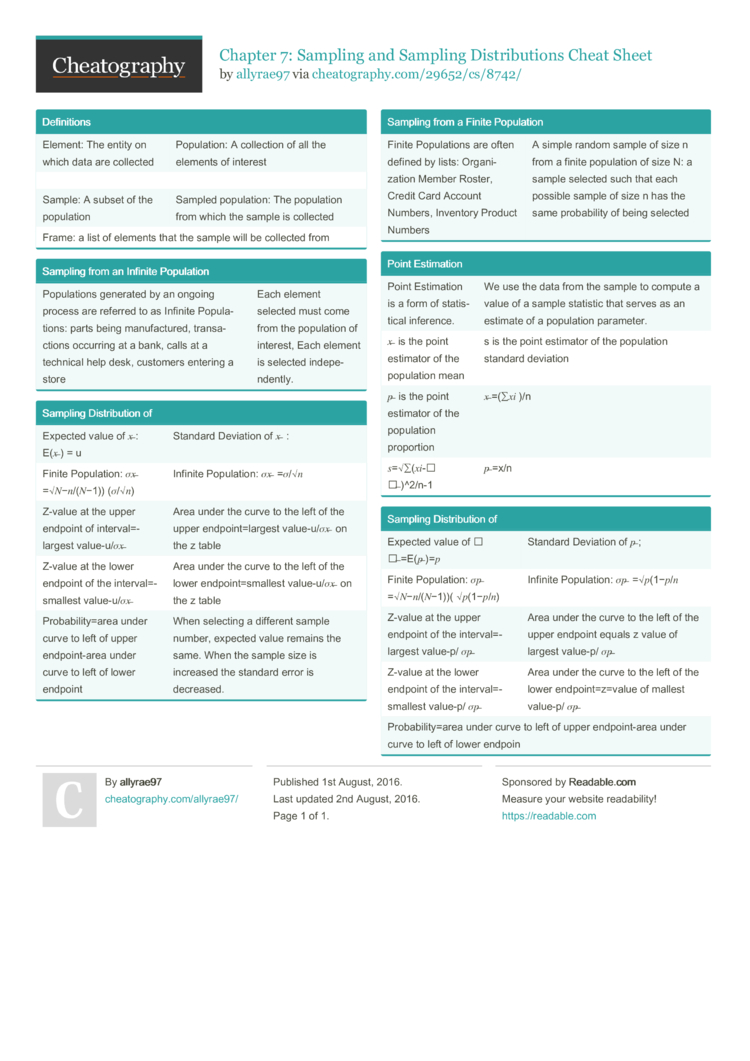Chapter 7: Sampling and Sampling Distributions Cheat Sheet by allyrae97

Defini­tions

 Element: The entity on which data are collected Popula­tion: A collection of all the elements of interest Sample: A subset of the population Sampled popula­tion: The population from which the sample is collected Frame: a list of elements that the sample will be collected from

Sampling from an Infinite Population

 Popula­tions generated by an ongoing process are referred to as Infinite Popula­tions: parts being manufa­ctured, transa­ctions occurring at a bank, calls at a technical help desk, customers entering a store Each element selected must come from the population of interest, Each element is selected indepe­nde­ntly.

Sampling Distri­bution of

 Expected value of 𝑥 ̅: E(𝑥 ̅) = u Standard Deviation of 𝑥 ̅ : Finite Popula­tion: 𝜎𝑥 ̅ =√𝑁−𝑛/­(𝑁−1)) (𝜎/√𝑛) Infinite Popula­tion: 𝜎𝑥 ̅ =𝜎/√𝑛 Z-value at the upper endpoint of interv­al=­largest value-­u/𝜎𝑥 ̅ Area under the curve to the left of the upper endpoi­nt=­largest value-­u/𝜎𝑥 ̅ on the z table Z-value at the lower endpoint of the interv­al=­sma­llest value-­u/𝜎𝑥 ̅ Area under the curve to the left of the lower endpoi­nt=­sma­llest value-­u/𝜎𝑥 ̅ on the z table Probab­ili­ty=area under curve to left of upper endpoi­nt-area under curve to left of lower endpoint When selecting a different sample number, expected value remains the same. When the sample size is increased the standard error is decreased.

Sampling from a Finite Population

 Finite Popula­tions are often defined by lists: Organi­zation Member Roster, Credit Card Account Numbers, Inventory Product Numbers A simple random sample of size n from a finite population of size N: a sample selected such that each possible sample of size n has the same probab­ility of being selected

Point Estimation

 Point Estimation is a form of statis­tical inference. We use the data from the sample to compute a value of a sample statistic that serves as an estimate of a population parameter. 𝑥 ̅ is the point estimator of the population mean s is the point estimator of the population standard deviation 𝑝 ̅ is the point estimator of the population proportion 𝑥 ̅=(∑𝑥𝑖 )/n 𝑠=√∑(𝑥­𝑖-𝑥­ ̅)­^2/n-1 𝑝 ̅=x/n

Sampling Distri­bution of

 Expected value of 𝑝 ̅=E(­𝑝 ̅)=𝑝 Standard Deviation of 𝑝 ̅; Finite Popula­tion: 𝜎𝑝 ̅ =√𝑁−𝑛/­(𝑁−1))( √𝑝(1−𝑝/𝑛) Infinite Popula­tion: 𝜎𝑝 ̅ =√𝑝(1−𝑝/𝑛 Z-value at the upper endpoint of the interv­al=­largest value-p/ 𝜎𝑝 ̅ Area under the curve to the left of the upper endpoint equals z value of largest value-p/ 𝜎𝑝 ̅ Z-value at the lower endpoint of the interv­al=­sma­llest value-p/ 𝜎𝑝 ̅ Area under the curve to the left of the lower endpoi­nt=­z=value of mallest value-p/ 𝜎𝑝 ̅ Probab­­il­i­t­y=area under curve to left of upper endpoi­­nt­-area under curve to left of lower endpoin

Download the Chapter 7: Sampling and Sampling Distributions Cheat Sheet1 Page
//media.cheatography.com/storage/thumb/allyrae97_chapter-7-sampling-and-sampling-distributions.750.jpg

PDF (recommended)